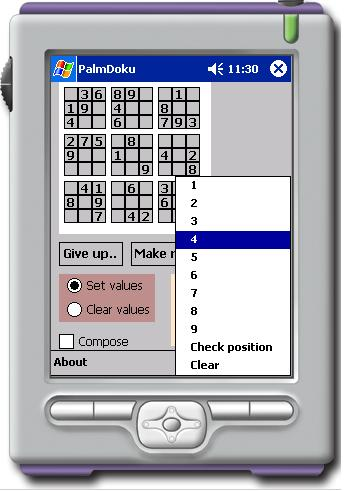Sudoku generating and solving using C# in PocketPC SDK

The sudoku game which sources can be downloaded above is a simple C# application created for pocketPC to show the use of Knuth's dancing links algorithm implementation to solve any sudoku puzzle.The main idea is to transform sudoku grid into a bitwise matrix as it is described in Knuth's lessons to use a simple algotirhm to find combination of lines in the matrix that create one line filled with 1's without repeating.

The interface is simple and created using a button array.

There are 2 main ideas implmented in the game :

1. Is a solver itself. (solver.cs - implementation of a solver class ).
2. How to generate a valid uniq solution sudoku puzzle.

In order to do so, I have used a few tricks to increase generation speed :

1. First I fill first 27 places (main diagonal - top left qubicle, middle qubicle and lower right qubicle with a random set of numbers 1..9, since there are no intersections it is quiet easy to do).
2. I use solver to find a first possible solution for a created grid.
3. I start removing numbers until I get a predefined number of clues left on grid while solution is unique.

If removing a number I get a non unique solution ( or solution can not be found) the number is put back and so on.